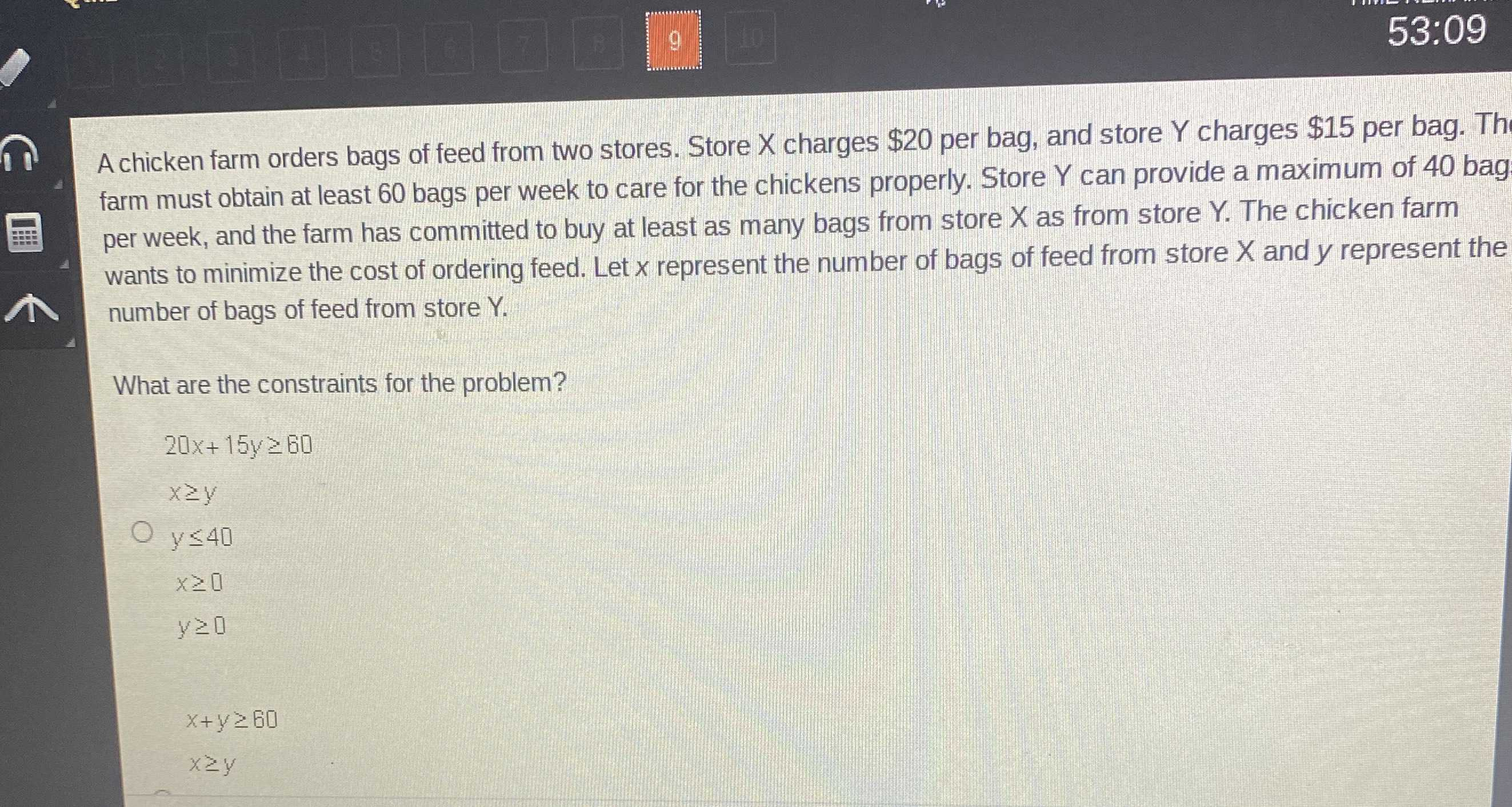### ¿Todavía tienes preguntas de matemáticas?

Pregunte a nuestros tutores expertos
Algebra
PreguntaA chicken farm orders bags of feed from two stores. Store $$X$$ charges $$\ 20$$ per bag, and store $$Y$$ charges $$\ 15$$ per bag. Th farm must obtain at least $$60$$ bags per week to care for the chickens properly. Store $$Y$$ can provide a maximum of $$40$$ bag per week, and the farm has committed to buy at least as many bags from store $$X$$ as from store $$Y$$ . The chicken farm wants to minimize the cost of ordering feed. Let $$x$$ represent the number of bags of feed from store $$X$$ and $$y$$ represent the number of bags of feed from store $$Y$$ . What are the constraints for the problem?

$$20 x + 15 y \geq 60$$

$$x \geq y$$

$$y \leq 40$$

$$x \geq 0$$

$$y \geq 0$$

X+Y$$\ge$$60
X$$\ge$$Y
Y$$\le$$40
Y$$\ge$$0
X$$> 0$$